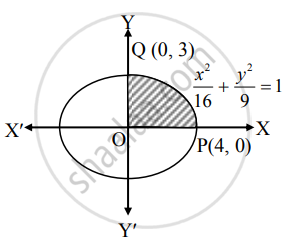# Solve the following : Find the area of the ellipse x216+y29 = 1. - Mathematics and Statistics

Sum

Solve the following :

Find the area of the ellipse x^2/(16) + y^2/(9) = 1.

#### Solution

By the symmetry of the ellipse, required area of the ellipse is 4 times the area of the region OPQO.
For the region OPQO, the limits of integration are x = 0 and x = 4.Given equation of the ellipse is x^2/(16) + y^2/(9) = 1

∴ y^2/(9) = 1 - x^2/(16)

∴ y2 = 9(1 - x^2/16)

= (9)/(16)(16 - x^2)

∴ y = ± (3)/(4)sqrt(16 - x^2)

∴ y = (3)/(4)sqrt(16 - x^2) ...[∵ In first quadrant, y > 0]

∴ Required area = 4(area of the region OPQO)

= 4int_0^4y*dx

= 4int_0^4 (3)/(4)sqrt(16 - x^2)*dx

= 3int_0^4 sqrt((4)^2 - x^2)*dx

= 3[x/2 sqrt((4)^2 - x^2) + (4)^2/(2)sin^-1 (x/4)]_0^4

= 3{[4/2 sqrt((4)^2 - (4)^2) + (4)^2/(2)sin^-1(4/4)] - [0/2 sqrt((4)^2 - (0)^2) + (4)^2/2sin^-1(0/4)]}

= 3{[0 + 8sin–1 (1)] – [0 + 0]}

= 3(8 xx pi/2)
= 12π sq. units.

Concept: Standard Forms of Ellipse
Is there an error in this question or solution?
Chapter 7: Applications of Definite Integration - Miscellaneous Exercise 7 [Page 158]

#### APPEARS IN

Balbharati Mathematics and Statistics 1 (Commerce) 12th Standard HSC Maharashtra State Board
Chapter 7 Applications of Definite Integration
Miscellaneous Exercise 7 | Q 4.4 | Page 158
Share